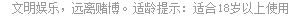# 憋七  当前版本: 2.0.1

#### 通过操作系统下载 - 最低系统版本 (安卓: 4.3; IOS: 8.0)>>1、玩牌规则

2、游戏规则

1、首轮规则

2、牌型说明

K 13 分， Q 12 分， J 11 分， 10 10 ……A 1

3、游戏术语

4、游戏规则

1、自己得分为每个人与自己的扣牌分差值的和。

A 5 B 8 C 1 D 12

A 的得分 =(B 扣牌分 -A 扣牌分 )+(C 扣牌分 -A 扣牌分 )+(D 扣牌分 -A 扣牌分 )

B 的得分 =(A 扣牌分 -B 扣牌分 )+(C 扣牌分 -B 扣牌分 )+(D 扣牌分 -B 扣牌分 )

C 的得分 =(A 扣牌分 -C 扣牌分 )+(B 扣牌分 -C 扣牌分 )+(D 扣牌分 -C 扣牌分 )

D 的得分 =(A 扣牌分 -D 扣牌分 )+(B 扣牌分 -D 扣牌分 )+(C 扣牌分 -D 扣牌分 )

A (8 - 5)+(1 - 5)+(12 - 5)=3 - 4 +7=6

B (5 - 8)+(1 - 8)+(12 - 8)=-3 -7 + 4=-6

C (5 - 1)+(8 - 1)+(12 - 1)=4 + 7 + 11=22

D (5 - 12)+(8 - 12)+(1 - 12) = -7 -4 -11=-22

2、当有一位用户走通时，其他三位用户的扣牌分都要加倍；当发生双通时，另两位用户的扣牌分都要乘以 4 ；当发生三通时，未通的一位用户扣牌分要乘以 8

3、每出现一张独头七，未通的每位用户的扣牌分要乘以 8

4007202233

## 官方QQ群

• 茶苑捕鱼：479257360    千变双扣：795175162
• 百变双扣：795642469    四副升级：795182559